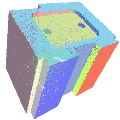CGAL 4.12.1 - Point Set Shape Detection
Point Set Shape Detection ReferenceSven Oesau, Yannick Verdie, Clément Jamin, Pierre Alliez, Florent Lafarge, Simon Giraudot
This component implements two primitive shape detection algorithms: efficient RANSAC and region growing. Planes are detected from point sets with unoriented normals. RANSAC also handles the following shapes: sphere, cylinder, cone and torus. Other types of shapes can be detected through implementing a class deriving from the base shape class.

Introduced in: CGAL 4.7
BibTeX: cgal:ovja-pssd-18b
Windows Demo: Polyhedron demo
Common Demo Dlls: dlls

## Concept

• ShapeDetectionTraits

## Main Classes

• CGAL::Shape_detection_3::Shape_detection_traits
• CGAL::Shape_detection_3::Efficient_RANSAC<Traits>
• CGAL::Shape_detection_3::Region_growing<Traits>

## Shape Interface

• CGAL::Shape_detection_3::Shape_base<Traits>

## Shape Classes

• CGAL::Shape_detection_3::Plane<Traits>
• CGAL::Shape_detection_3::Sphere<Traits>
• CGAL::Shape_detection_3::Cylinder<Traits>
• CGAL::Shape_detection_3::Cone<Traits>
• CGAL::Shape_detection_3::Torus<Traits>

## Functions

• CGAL::regularize_planes()

## Property Maps

• CGAL::Shape_detection_3::Plane_map<Traits>
• CGAL::Shape_detection_3::Point_to_shape_index_map<Traits>

Concepts

Shapes

## Files

file  Shape_detection_3.h

file  regularize_planes.h

## Classes

class  CGAL::Shape_detection_3::Efficient_RANSAC< Traits >
A shape detection algorithm using a RANSAC method. More...

class  CGAL::Shape_detection_3::Region_growing< Traits >
A shape detection algorithm using a region growing method. More...

struct  CGAL::Shape_detection_3::Shape_detection_traits< Gt, InputRange, InputPointMap, InputNormalMap >
Default traits class to use the shape detection class Efficient_RANSAC. More...

class  CGAL::Shape_detection_3::Point_to_shape_index_map< Traits >
Property map that associate a point index to its assigned shape found by a shape detection algorithm (such as CGAL::Shape_detection_3::Efficient_RANSAC or CGAL::Shape_detection_3::Region_growing). More...

class  CGAL::Shape_detection_3::Plane_map< Traits >
Property map that associates a detected plane object (CGAL::Shape_detection_3::Plane) to a CGAL::Plane_3 object. More...

## Functions

template<typename PointRange , typename PointMap , typename PlaneRange , typename PlaneMap , typename IndexMap , typename Kernel >
void CGAL::regularize_planes (const PointRange &points, PointMap point_map, PlaneRange &planes, PlaneMap plane_map, IndexMap index_map, const Kernel &, bool regularize_parallelism, bool regularize_orthogonality, bool regularize_coplanarity, bool regularize_axis_symmetry, double tolerance_angle=25.0, double tolerance_coplanarity=0.01, typename Kernel::Vector_3 symmetry_direction=typename Kernel::Vector_3(0., 0., 1.))
Given a set of detected planes with their respective inlier sets, this function enables to regularize the planes: More...

## ◆ regularize_planes()

template<typename PointRange , typename PointMap , typename PlaneRange , typename PlaneMap , typename IndexMap , typename Kernel >
 void CGAL::regularize_planes ( const PointRange & points, PointMap point_map, PlaneRange & planes, PlaneMap plane_map, IndexMap index_map, const Kernel & , bool regularize_parallelism, bool regularize_orthogonality, bool regularize_coplanarity, bool regularize_axis_symmetry, double tolerance_angle = 25.0, double tolerance_coplanarity = 0.01, typename Kernel::Vector_3 symmetry_direction = typename Kernel::Vector_3 (0., 0., 1.) )

#include <CGAL/regularize_planes.h>

Given a set of detected planes with their respective inlier sets, this function enables to regularize the planes:

• Planes near parallel can be made exactly parallel.
• Planes near orthogonal can be made exactly orthogonal.
• Planes parallel and near coplanar can be made exactly coplanar.
• Planes near symmetrical with a user-defined axis can be made exactly symmetrical.

Planes are directly modified. Points are left unaltered, as well as their relationships to planes (no transfer of point from a primitive plane to another).

The implementation follows .

Template Parameters
 PointRange range of points, model of ConstRange PointPMap is a model of ReadablePropertyMap with value type Kernel::Point_3. It can be omitted if the value type of the iterator of PointRange is convertible to Point_3. PlaneRange range of planes, model of Range PlaneMap is a model of WritablePropertyMap with value type Kernel::Plane_3. It can be omitted if the value type of the iterator of PlaneRange is convertible to Plane_3. IndexMap is a model of ReadablePropertyMap with value type int. Kernel Geometric traits class. It can be omitted and deduced automatically from the value type of PointMap.
Parameters
 points range of points. point_map property map: value_type of typename PointRange::const_iterator -> Point_3 planes range of planes. plane_map property map: value_type of typename PlaneRange::iterator -> Plane_3 index_map property map: index of point std::size_t -> index of plane int (-1 if the point is not assigned to a plane) regularize_parallelism Select whether parallelism is regularized or not. regularize_orthogonality Select whether orthogonality is regularized or not. regularize_coplanarity Select whether coplanarity is regularized or not. regularize_axis_symmetry Select whether axis symmetry is regularized or not. tolerance_angle Tolerance of deviation between normal vectors of planes (in degrees) used for parallelism, orthogonality and axis symmetry. Default value is 25 degrees. tolerance_coplanarity Maximal distance between two parallel planes such that they are considered coplanar. Default value is 0.01. symmetry_direction Chosen axis for symmetry regularization. Default value is the Z axis.
Examples:
Point_set_shape_detection_3/plane_regularization.cpp.